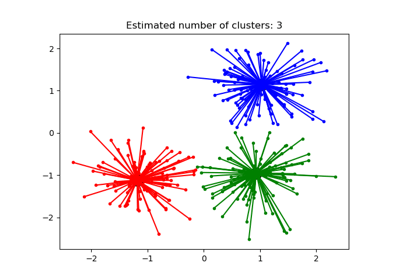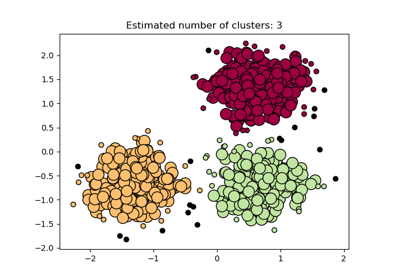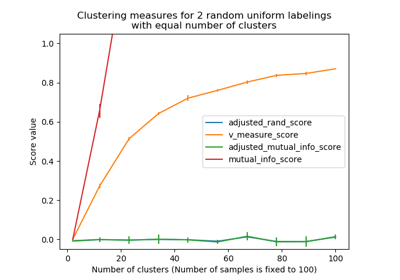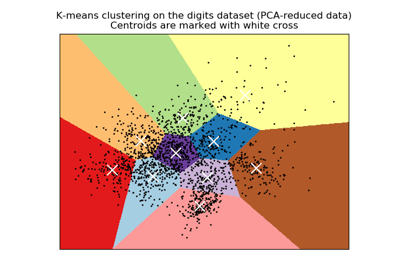# W3cubDocs

/scikit-learn

`sklearn.metrics.adjusted_mutual_info_score(labels_true, labels_pred, average_method=’warn’)` [source]

Adjusted Mutual Information between two clusterings.

Adjusted Mutual Information (AMI) is an adjustment of the Mutual Information (MI) score to account for chance. It accounts for the fact that the MI is generally higher for two clusterings with a larger number of clusters, regardless of whether there is actually more information shared. For two clusterings \(U\) and \(V\), the AMI is given as:

```AMI(U, V) = [MI(U, V) - E(MI(U, V))] / [avg(H(U), H(V)) - E(MI(U, V))]
```

This metric is independent of the absolute values of the labels: a permutation of the class or cluster label values won’t change the score value in any way.

This metric is furthermore symmetric: switching `label_true` with `label_pred` will return the same score value. This can be useful to measure the agreement of two independent label assignments strategies on the same dataset when the real ground truth is not known.

Be mindful that this function is an order of magnitude slower than other metrics, such as the Adjusted Rand Index.

Read more in the User Guide.

Parameters: `labels_true : int array, shape = [n_samples]` A clustering of the data into disjoint subsets. `labels_pred : array, shape = [n_samples]` A clustering of the data into disjoint subsets. `average_method : string, optional (default: ‘warn’)` How to compute the normalizer in the denominator. Possible options are ‘min’, ‘geometric’, ‘arithmetic’, and ‘max’. If ‘warn’, ‘max’ will be used. The default will change to ‘arithmetic’ in version 0.22. New in version 0.20. ami: float (upperlimited by 1.0) The AMI returns a value of 1 when the two partitions are identical (ie perfectly matched). Random partitions (independent labellings) have an expected AMI around 0 on average hence can be negative.

`adjusted_rand_score`
`mutual_info_score`
Mutual Information (not adjusted for chance)

#### Examples

Perfect labelings are both homogeneous and complete, hence have score 1.0:

```>>> from sklearn.metrics.cluster import adjusted_mutual_info_score
>>> adjusted_mutual_info_score([0, 0, 1, 1], [0, 0, 1, 1])
...
1.0
>>> adjusted_mutual_info_score([0, 0, 1, 1], [1, 1, 0, 0])
...
1.0
```

If classes members are completely split across different clusters, the assignment is totally in-complete, hence the AMI is null:

```>>> adjusted_mutual_info_score([0, 0, 0, 0], [0, 1, 2, 3])
...
0.0
```

## Examples using `sklearn.metrics.adjusted_mutual_info_score`Demo of affinity propagation clustering algorithmDemo of DBSCAN clustering algorithmAdjustment for chance in clustering performance evaluationA demo of K-Means clustering on the handwritten digits data